# RS Aggarwal Class 9 Solutions Chapter 5 - Congruence And Inequalities In Triangle Ex 5B (5.2)

## RS Aggarwal Class 9 Chapter 5 – Congruence And Inequalities In Triangle Ex 5B (5.2) Solutions Free PDF

The RS Aggarwal Class 9 solution helps students in making the foundation strong and offering them with countless questions to practice. So, to assist you in solving the various questions, we at BYJU’S providing the RS Aggarwal Class 9 solutions for maths which are drafted by our subject experts who hold huge academic experience. Our subject experts make extra effort to put the best possible information with each explanation for different topics.

With the help of these solutions, you can not only crack the exam but can also obtain the best score in the exams. The easy to understand concepts, step by step solving of the questions help students in the preparation of the exam. The RS Aggarwal Class 9 Solutions Chapter 5 – Congruence And Inequalities In Triangle Ex 5B (5.2) help you in anticipating the type of questions which can be asked in the exam.

## Download PDF of RS Aggarwal Class 9 Solutions Chapter 5 – Congruence And Inequalities In Triangle Ex 5B (5.2)

Question 1:

In the given figure, if x = y and AB = CB, then prove that AE = CD.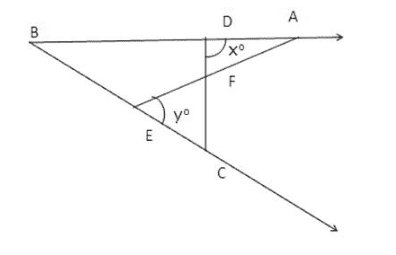Solution:

Given: AB = BC

and, xo = yo

To prove: AE = CD

Proof: In $\Delta$ABE, we have,

Exterior $\angle$AEB = $\angle$EBA + $\angle$BAE

$\Rightarrow$ yo = $\angle$EBA + $\angle$BAE

Again, in $\Delta$BCD, we have

xo = $\angle$CBA + $\angle$BCD

Since, xo = yo [Given]

So, $\angle$EBA + $\angle$BAE = $\angle$CBA + $\angle$BCD

$\Rightarrow$ $\angle$BAE = $\angle$BCD

Thus in $\Delta$BCD and $\Delta$BAE, we have

$\angle$B = $\angle$B [Common]

BC = AB [Given]

and, \) $\angle$BAE = $\angle$BCD [Proved above]

Thus by Angle-Side-Angle criterion of congruence, we have

$\Delta$BCD $\cong$ $\Delta$BAE

The corresponding parts of the congruent triangles are equal.

So, CD = AE [Proved]

Question 2:

ABC is a triangle in which AB = AC. If the bisectors of $\angle$B and $\angle$C meet AC and AB in D and E respectively, prove that BD = CE.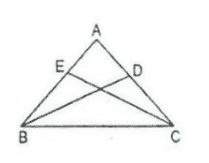Solution:

Given: A $\Delta$ABC in which AB = AC and BD and CE are the bisectors of $\angle$B and $\angle$C respectively.

To prove: BD = CE

Proof: In $\Delta$ABC and $\Delta$ACE

$\angle$ABD = ½ $\angle$B

and, $\angle$ACE = ½ $\angle$C

But $\angle$B = $\angle$C as AB = AC [In isosceles triangle, base angles are equal]

$\Rightarrow$ $\angle$ABD = $\angle$ACE

AB = AC [Given]

$\angle$A = $\angle$A [Common]

Thus by Angle-Side-Angle criterion of congruence, we have

$\Delta$ABD $\cong$ $\Delta$ACE

The corresponding parts of the congruent triangles are equal.

BD = CE [c.p.c.t]

Question 3:

In the adjoining figure, AD is a median of $\Delta$ABC. If BL and CM are drawn perpendiculars on AD and AD produced, prove that BL = CM.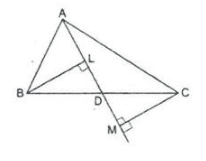Solution:

Given: A triangle in which D is the midpoint of BC and BL $\perp$ AD and CM $\perp$ AD

To prove: BL = CM

Proof: In $\Delta$BLD and $\Delta$CMD

$\angle$BLD = $\angle$CMD = 90o [Given]

$\angle$BDL = $\angle$MDC [Vertically opposite angles]

BD = DC [Given]

Thus by Angle-Angle-Side criterion of congruence, we have

$\Delta$BLD $\cong$ $\Delta$CMD

The corresponding parts of the congruent triangles are equal

So, BL = CM [c.p.c.t]

Question 4:

In $\Delta$ABC, D is the mid-point of BC. If DL $\perp$ AB and DM $\perp$ AC such that DL = DM, prove that AB = AC.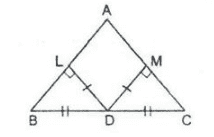Solution:

Given: In a $\Delta$ABC, D is the midpoint of BC and DL $\perp$ AB and DM $\perp$ AC. Also, DL = DM

To prove: AB = AC

Proof: In right angled triangle $\Delta$BLD and $\Delta$CMD

$\angle$BLD = $\angle$CMD = 90o

Hypt. BD = Hypt. CD [Given]

DL = DM [Given]

Thus, by Right Angle-Hypotenuse_side criterion of congruence, we have

$\Delta$BLD $\cong$ $\Delta$CMD

The corresponding parts of the congruent triangles are equal.

Therefore, $\angle$ABD = $\angle$ACD [c.p.c.t]

In $\Delta$ABC, we have

$\angle$ABD = $\angle$ACD

$\Rightarrow$ AB = AC [Since opposite to equal angles are equal]

Question 5:

In $\Delta$ABC, AB = AC and the bisectors of $\angle$B and $\angle$C meet at a point O. Prove that BO = CO and the ray AO is the bisector of $\angle$A.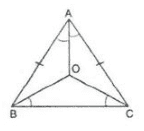Solution:

Given: A $\Delta$ABC in which AB = AC, BO and CO are bisectors of $\angle$B and $\angle$C

To prove: In $\Delta$BOC, we have,

$\angle$OBC = ½ $\angle$B

and, $\angle$OCB = ½ $\angle$C

But, $\angle$B = $\angle$C [therefore AB = AC (given)]

So, $\angle$OBC = $\angle$OCB

Since base angles are equal, sides are equal

$\Rightarrow$ OB = OC ——- (1)

Since OB and OC are the bisectors of angles,

$\angle$B and $\angle$C respectively, we have

$\angle$ABO = ½ $\angle$B

$\angle$ACO = ½ $\angle$C

$\Rightarrow$ $\angle$ABO = $\angle$ACO  ——— (2)

Now, in $\Delta$ABO and $\Delta$ACO

AB = AC [Given]

$\angle$ABO = $\angle$ACO [from (2)]

BO = OC [from(1)]

Thus, by Side-Angle-Side criterion of congruence, we have

$\Delta$ABO $\cong$ $\Delta$ACO

The corresponding parts of the congruent triangles are equal

Therefore, $\angle$BAO = $\angle$CAO [by c.p.c.t]

i.e. , AO bisects $\angle$A.

Question 6:

In the given figure, PQR is an equilateral triangle and QRST is a square. Prove that

(i) PT = PS

(ii) $\angle$PSR = 15o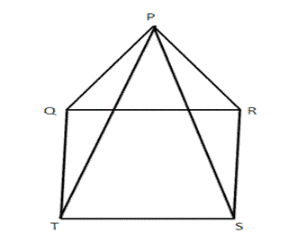Solution:

Given: PQR is an equilateral triangle and QRST is a square.

To prove: PT = PS

and $\angle$PSR = 15o

Proof: Since $\Delta$ is an equilateral triangle,

$\angle$ = 60o and $\angle$PRQ = 60o

Since QRTS is a square,

$\angle$RQT = 90o and $\angle$QRS = 90o

In $\Delta$PQT

$\angle$PQT = $\angle$PQR + $\angle$RQT

= 60o + 90o = 150o

In $\Delta$PRS

$\angle$PRS = $\angle$PRQ + $\angle$QRS

=60o + 90o = 150o ——- (1)

$\Rightarrow$ $\angle$PQT = $\angle$PRS ——— (2)

Thus, in $\Delta$PQT and $\Delta$PRS

PQ = RS [sides of equilateral triangle PQR]

$\angle$PQT = $\angle$PRS ——– (2)

QT = RS [sides of square QRST]

Thus, by Side-Angle-Side criterion of congruence, we have

therefore,  $\Delta$PQT $\cong$ $\Delta$PRS

The corresponding parts of the congruent triangles are equal.

Therefore, PT = PS [c.p.c.t]

Now in $\Delta$PRS, we have

PR = RS

$\Rightarrow$ $\angle$ RPS = $\angle$PSR

But $\angle$PRS = 150o [from(1)]

So, by angle sum property in $\Delta$PRS

$\angle$PRS + $\angle$SPR + $\angle$PSR = 180o

$\Rightarrow$ 150o + $\angle$PSR + $\angle$PSR = 180o

$\Rightarrow$ 2$\angle$PSR = 180o – 150o

$\Rightarrow$ $\angle$PSR = 30o/2 = 15o

Question 7:

In the given figure, ABC is a triangle, right angled at B. If BCDE is a square on side BC and ACFG is a square on AC, prove that AD = BF.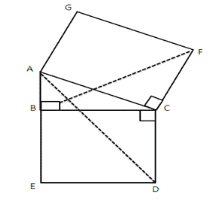Solution:

Given: ABC is a triangle right angled at B. ACFG is a square and BCDE is a square.

To prove: AD = EF

Proof: Since BCDE is a square,

$\angle$BCD = 90o ——- (1)

In $\Delta$ACD,

$\angle$ACD = $\angle$ACB + $\angle$BCD

= $\angle$ACB + 90o ——- (2)

In $\Delta$BCF,

$\angle$BCF = $\angle$BCA + $\angle$ACF

Since ACFG is a square,

$\angle$ACF = 90o

Thus, we have

$\angle$BCF = $\angle$BCA + 90o ——- (3)

From (2) and (3), we have

$\angle$ACD = $\angle$BCF ——— (4)

Thus in $\Delta$ACD and $\Delta$BCF, we have

AC = CF [sides of a square]

$\angle$ACD = $\angle$BCF [from (4)]

CD = BC [sides of a square]

Thus, by Side-Angle-Side criterion of congruence, we have

Therefore, $\Delta$ACD $\cong$ $\Delta$BCF

The corresponding parts of congruent triangles are equal.

So, AD = BF (c.p.c.t)

Question 8:

Prove that the median from the vertex of an isosceles triangle is the bisector of the vertical angle.

Solution:

Given: ABC is a isosceles triangle in which AB = AC and AD is the median through A.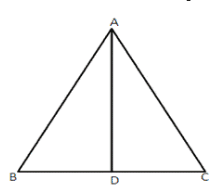To prove: $\angle$BAD = $\angle$DAC

Proof: In $\Delta$ABD and $\Delta$ADC

AB = AC [Given]

BD = DC [Given]

Thus by Side-Side-Side criterion of congruence, we have

$\Delta$ABD $\cong$ $\Delta$ADC

The corresponding parts of the congruent triangles are equal.

Therefore, $\angle$BAD = $\angle$DAC (Proved)

Question 9:

In the given figure, ABCD is quadrilateral in which AB || DC and P is the mid-point of BC. On producing, AO and DC meet at Q. Prove that

(i) AB = CQ

(ii) DQ = DC + AB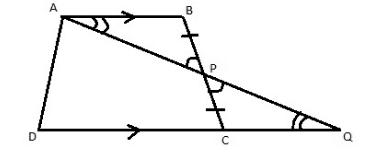Solution:

Given ABCD is a quadrilateral in which AB || DC

To prove:

i) AB = CQ

ii) DQ = DC + AB

Proof: In $\Delta$ABP and $\Delta$PCQ we have

$\angle$PAB = $\angle$PQC [alternate angles]

$\angle$APB = $\angle$CPQ [vertically opposite angles]

BP = PC [Given]

Thus by Angle-Angle-Side criterion of congruence, we have

$\Delta$ABP $\cong$ $\Delta$PCQ

The corresponding parts of the congruent triangles are equal

Therefore, AB = CQ ——– (1)

Now, DQ = DC + CQ

= DC + AB [from (1)]

Question 10:

In the given figure, OA = OB and OP = OQ. Prove that

(i) PX = QX

(ii) AX = BX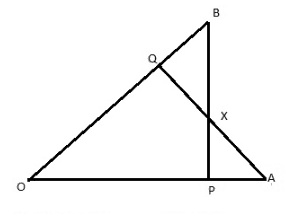Solution:

Given: OA = OB and OP = OQ

To prove:

(i) PX = QX

(ii) AX = BX

Proof: In $\Delta$OAQ and $\Delta$OPB, we have,

OA = OB [Given]

$\angle$O = $\angle$O [Common]

OQ = OP [Given]

Thus by Side-Angle-Side criterion of congruence, we have

$\Delta$OAQ $\cong$ $\Delta$OPB

The corresponding parts of the congruent triangles are equal.

Therefore, $\angle$OBP = $\angle$OAQ ——— (1)

Thus, in $\Delta$BXQ and $\Delta$PXA, we have

BQ = OB – OQ

and, PA = OA – OP

But, OP = OQ

and OA =OB [Given]

Therefore, we have, BQ = PA ———- (2)

Now consider triangles $\Delta$BXQ and $\Delta$PXA.

$\angle$BXQ = $\angle$PXA [vertically opposite angles]

$\angle$OBP = $\angle$OAQ [from (1)]

BQ = PA [from (2)]

Thus by Angle-Angle-Side criterion of congruence, we have,

Therefore, $\Delta$BXQ $\cong$ $\Delta$PPXA

PX = QX [c.p.c.t]

AX = BX [c.p.c.t]’

### Key Features of RS Aggarwal Class 9 Solutions Chapter 5 – Congruence And Inequalities In Triangle Ex 5B (5.2)

• It is the best reference material to practice different types of questions.
• The solutions help students to be confident while solving the final question paper.
• It improves your speed and accuracy.
• It analyzes your performance and preparation level.